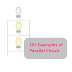Here is the list of common examples of parallel circuit,
1. House lighting circuit
2. Streetlight Circuit
3. Conventional Fire Alarm Circuit
4. LC Tank Circuit
5. Power Socket or Outlet
6. DC Filter Circuit
7. Electrical Power Distribution Circuit
8. Current Divider Circuit
9. Speaker Connection in large audio system
10. Power Factor Correction Circuit
11. Noise Filtering Circuit

Parallel circuits are those where all the components are connected in parallel to each other. Here, the voltage across all the components is the same but the flow of current through individual components is not the same, it depends upon their internal resistance. So the characteristics of a parallel circuit exact opposite of a series circuit.

Now, let's discuss about the above example so it will give a clear knowledge about parallel circuits. The first one is the house lighting circuit. All the lights even all other loads in a house or office are connected in parallel to each other. In the USA the utility supply for a home is 120V, and in India is 230V. Also, we know that all the loads including lights at our homes are have the same voltage rating. So they are connected in parallel to maintain the rated utility supply voltage across all the loads.

Streetlights are also connected in parallel. And we know that in a parallel circuit damaging any one load cannot affect the other loads. So if any one street light got damaged, other lights will without any interruption. Also, note that as the voltage across each light is equal so the brightness also be the same.

Another example of a parallel circuit is LC tank Circuit. LC tank circuit is the parallel combination of an inductor and a capacitor. The function of an LC tank circuit is to generate an oscillating or dumping signal.

Power sockets or outlets in our power distribution boards are connected in parallel to each other so we can get the same voltage, current, and power from each socket or outlet. Generally, the phase terminal of each socket is connected together. Same as the neutral terminal of each socket is connected together. And ground or earthing terminal of each socket is connected together. If there is any wrong connection between phase and neutral, the polarity of the power supply will be changed.

We know that condensers or capacitors are connected in parallel with the inductive load to correct the power factor. So it is an example of a parallel circuit. basically, the condenser consumed the reactive power to keep the power factor near unity.

In large audio systems such as PA systems, concerts, and theaters all the speakers are connected in parallel. Even the polarity of the connection is also maintained correctly to get a better sound experience. So the speaker connection in parallel is also an example of a parallel circuit.

Parallel Circuit Application and Uses

1. A parallel circuit is used to maintain the same voltage level across all the loads and components connected to it.
2. A parallel circuit is used to eliminate the effect of damaging anyone's load to the other as in the series circuit.
3. A parallel circuit is very helpful to operate multiple loads with a small voltage.

Parallel Circuit Examples and ApplicationsReviewed by Author on 5/13/2022 Rating: 5